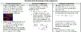# FactsWise Multiply Divide Basic Facts Resource BookSubject
Resource Type
File Type

PDF

(16 MB|107 pages)
Standards
• Product Description
• StandardsNEW

FactsWise is a research-based and researched program focused on developing basic facts fluency. This book provides activities to help students learn their facts, and at the same time to develop their part-whole thinking. The book also includes correlations to Common Core math standards, and help for teachers who want to learn more about strategies using properties of operations.

This August 2016 edition of the FactsWise Multiplication/Division Resource Book includes new formative assessment tools and the Goal 1, 2 and 5 Activity Progressions.

Fluently multiply and divide within 100, using strategies such as the relationship between multiplication and division (e.g., knowing that 8 × 5 = 40, one knows 40 ÷ 5 = 8) or properties of operations. By the end of Grade 3, know from memory all products of two one-digit numbers.
Understand division as an unknown-factor problem. For example, find 32 ÷ 8 by finding the number that makes 32 when multiplied by 8.
Apply properties of operations as strategies to multiply and divide. Examples: If 6 × 4 = 24 is known, then 4 × 6 = 24 is also known. (Commutative property of multiplication.) 3 × 5 × 2 can be found by 3 × 5 = 15, then 15 × 2 = 30, or by 5 × 2 = 10, then 3 × 10 = 30. (Associative property of multiplication.) Knowing that 8 × 5 = 40 and 8 × 2 = 16, one can find 8 × 7 as 8 × (5 + 2) = (8 × 5) + (8 × 2) = 40 + 16 = 56. (Distributive property.)
Determine the unknown whole number in a multiplication or division equation relating three whole numbers. For example, determine the unknown number that makes the equation true in each of the equations 8 × ? = 48, 5 = __ ÷ 3, 6 × 6 = ?.
Use multiplication and division within 100 to solve word problems in situations involving equal groups, arrays, and measurement quantities, e.g., by using drawings and equations with a symbol for the unknown number to represent the problem.
Total Pages
107 pages
N/A
Teaching Duration
Lifelong tool
Report this Resource to TpT
Reported resources will be reviewed by our team. Report this resource to let us know if this resource violates TpT’s content guidelines.